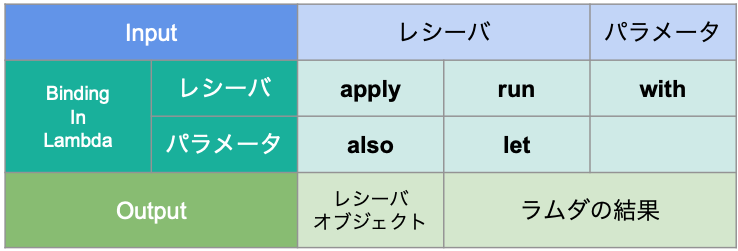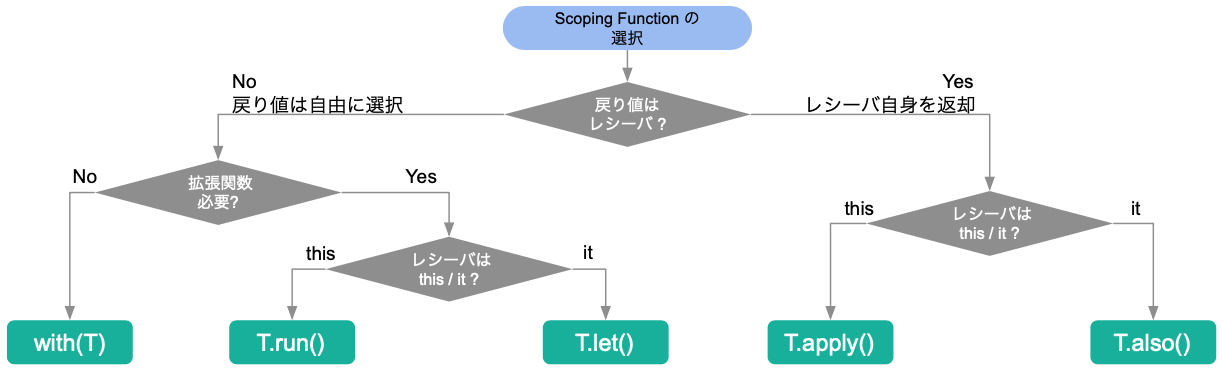# [JAVA] With Kotorin ―― 7. Scoping Function

## Overview / Description

In "Spring with Kotorin-- 6. Asynchronous processing", the following description was made when setting the property of the instance. It was.

``````    @Bean
corePoolSize = 1
setQueueCapacity(5)
maxPoolSize = 1
}
``````

It creates a ThreadPoolTaskExecutor instance and then lambda operates on that instance.

In this way, the function used to operate in lambda following the instance is called ** scope function **. Using this format is convenient in the following points.

--Operations to instances can be grouped in a lambda --Eliminate code redundancy --The range of influence of the operation can be contained only in the lambda

Kotlin has multiple scope functions. Each one is used differently, so let's check what it looks like.

## Assumptions / Environment

It is implemented in the following environment, but it is not a prerequisite.

### Runtime version

• Kotlin : 1.3.0
• SpringBoot : 2.1.0.RELEASE

Spring Dependencies

• Web
• Actuator

### Development environment

• OS : Mac
• IDE : IntelliJ IDEA

## Procedure / Explanation

The scope functions are as follows.

• with
• run
• let
• apply
• also

Let's look at each function.

### Definition of scope function

First, let's look at the definition of each scope function.

Scoping Function Definition Example
with fun <T, R> with(receiver: T, block: T.() -> R): R = receiver.block() val r: R = with(T()) { this.foo(); this.toR() }
run fun <T, R> T.run(block: T.() -> R): R = block() val r: R = T().run { this.foo(); this.toR() }
let fun <T, R> T.let(block: (T) -> R): R = block(this) val r: R = T().let { it.foo(); it.toR() }
apply fun T.apply(block: T.() -> Unit): T { block(); return this } val t: T = T().apply { this.foo() }
also fun T.also(block: (T) -> Unit): T { block(this); return this } val t: T = T().also { it.foo() }

Looking at the definition, you can see that the definition method is largely different from the following two viewpoints.

--Representation of receiver --`T. ()-> R`: Receiver type T extension function --`(T)-> R`: Handle receiver type T as an argument

• Return value --`R = block () `: Return the result of lambda --`return this`: Return the receiver

Summarizing the above, we have the following matrix.### Code sample

Prepare the following data class as a receiver object.

``````data class Person(
var name: String,
var age: Int
) {
private lateinit var hobby: String
fun nameToUpperCase() {
name = name.toUpperCase()
}

fun increaseAge() {
age++
}

fun defineHobby(hobby: String) {
this.hobby = hobby
}

fun displayHobby() = this.hobby

return "Person(name=\$name, age=\$age, hobby=\$hobby)"
}
}
``````

with `with` is an ordinary function, not an extension function of the receiver. Therefore, it is called differently from other scope functions.

``````    val foo: Person = Person("with", 20)

with(foo){
foo.increaseAge()
println(foo)
}
``````

run `run` uses` this` to represent the receiver in the lambda. Therefore, it is ** optional **. In the following, it is explicitly described, but it is often omitted because it is redundant. It also returns the lambda result (last line).

``````    val foo: Person = Person("run", 20)

return foo.run {
nameToUpperCase()
println(this)
this.name
}
``````

let `let` behaves much like run, but The receiver representation in the lambda is ʻit`. Therefore, it can be ** alias **. Replace it with a keyword that makes sense It is used to improve readability.

``````    val foo: Person = Person("let", 20)

return foo.let { it ->
println(it)
it.defineHobby("Kotlin")
it.displayHobby()
}
``````

apply ʻApply`returns the receiver itself. Therefore, it is used when operating the receiver itself. It is used for many purposes such as setting and changing properties. The receiver is represented by`this`. Here, it is omitted in the function call of the receiver.

``````    val foo: Person = Person("apply", 20)

foo.apply {
println(this)
nameToUpperCase()
increaseAge()
println(this)
}
``````

also You can think of ʻalso` as the receiver representation of apply is now ʻit`. Therefore, it is used when it is necessary to give an alias to the receiver to improve readability.

``````    val foo: Person = Person("also", 20)

return foo.also { it ->
println(it)
it.nameToUpperCase()
it.increaseAge()
it.defineHobby("Kotlin")